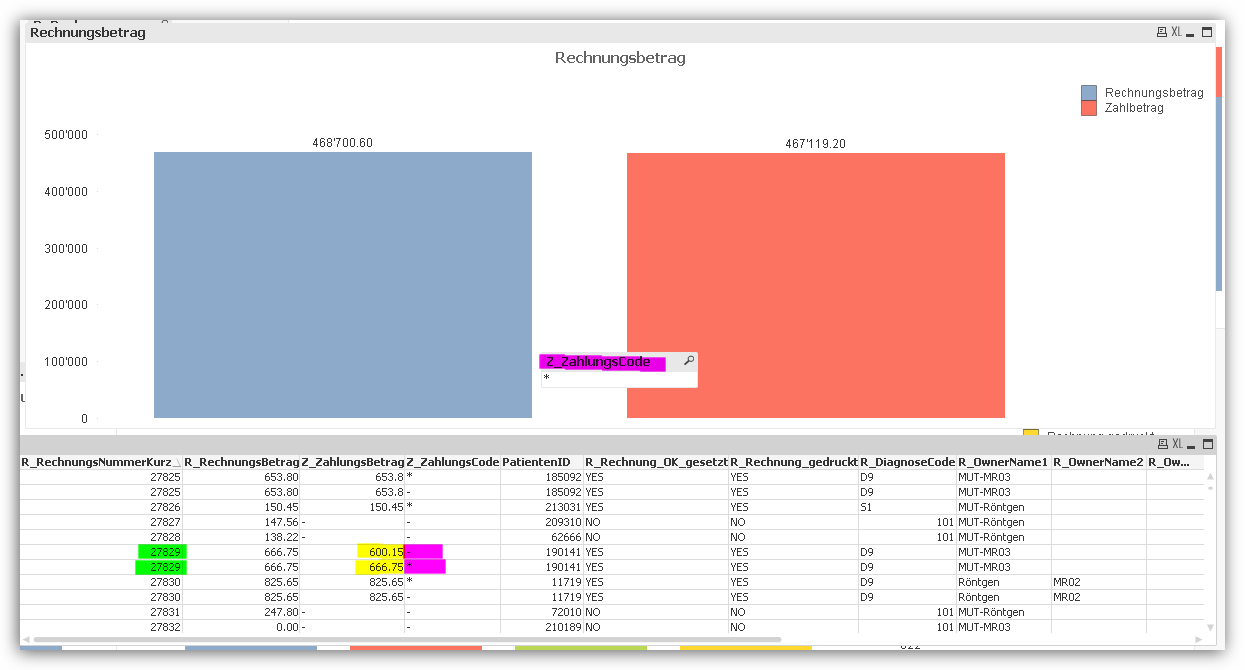# New to QlikView

Discussion board where members can get started with QlikView.

Announcements
Leverage your QlikView investment to modernize BI – see how! Join Group
cancel
Showing results for
Did you mean:
HighlightedNot applicable

## Selecting NULL-Values

Hello everybody

I have some Problems with a SetAnalysis Expression.

I try to select values based on a field (Z_ZahlungsCode) that only contains two values. These values are NULL or the character "*".

If I use this expression:

Sum ({\$<[R_Debitor_abgschlossen]={"YES"}, [Z_ZahlungsCode]={"*"}>}[Z_ZahlungsBetrag])

I get the the correct results (the sum of all records where Z_Zahlungscode is *)

But actually I want it the other way around. I would like to have the sum of all records with the NULL-Value. I tried different things like

Sum ({\$<[R_Debitor_abgschlossen]={"YES"}, [Z_ZahlungsCode]={""}>}[Z_ZahlungsBetrag])

Sum ({\$<[R_Debitor_abgschlossen]={"YES"}, [Z_ZahlungsCode]=NULL>}[Z_ZahlungsBetrag])

Sum ({\$<[R_Debitor_abgschlossen]={"YES"}, [Z_ZahlungsCode]-={"*"}>}[Z_ZahlungsBetrag])

Sum ({\$<[R_Debitor_abgschlossen]={"YES"}, [Z_ZahlungsCode]=0>}[Z_ZahlungsBetrag])

But all these variations did not work. The result was allways Sum = 0

Does anybody have an idea?

Thanks a lot

Achim

Tags (3)
5 Replies
HighlightedMVP

## Re: Selecting NULL-Values

May be this:

Sum({\$<[R_Debitor_abgschlossen]={"YES"}, [Z_ZahlungsCode]={"=NullCount([Z_ZahlungsCode]) > 0"}>}[Z_ZahlungsBetrag])

or

Sum({\$<[R_Debitor_abgschlossen]={"YES"}, [Z_ZahlungsCode]={"=Len(Trim([Z_ZahlungsCode])) = 0"}>}[Z_ZahlungsBetrag])

or

Sum({\$<[R_Debitor_abgschlossen]={"YES"}, [Z_ZahlungsCode]={"=IsNull([Z_ZahlungsCode])"}>}[Z_ZahlungsBetrag])

HighlightedMVP

## Re: Selecting NULL-Values

I would suggest using something like

Sum ({\$<[R_Debitor_abgschlossen]={"YES"}, KeyField = e({< [Z_ZahlungsCode]={"*"}>}) >} [Z_ZahlungsBetrag])

Excluding values in Set Analysis

HighlightedNot applicable

## Re: Selecting NULL-Values

Hello Sunny

Thank you for your help. Unfortunatly this does not work.

Maybe it is an issue of the database and the datamodel that I use. As you can see below. I have two records for each bill (bill number in first colum, highlighted in green). One record stands for the sum on the bill (highlighted in yellow) indicated bei the *(highlighted in pink). I can calculate a sum on these records with the * (see Chart, red bar). I also opend a list box to filter the field "Z_Zahlungscode". But if you can see, there ist only the * that I can select. In the table below the "NULL"-Values seem to be indicated bei a "-".Maybe you have another idea.

Achim

HighlightedNot applicable

## Re: Selecting NULL-Values

Hello

Thank you for your help. Unfortunatly this does not work.

Maybe it is an issue of the database and the datamodel that I use. As you can see below. I have two records for each bill (bill number in first colum, highlighted in green). One record stands for the sum on the bill (highlighted in yellow) indicated bei the *(highlighted in pink). I can calculate a sum on these records with the * (see Chart, red bar). I also opend a list box to filter the field "Z_Zahlungscode". But if you can see, there ist only the * that I can select. In the table below the "NULL"-Values seem to be indicated bei a "-".

Maybe you have another idea.

AchimHighlightedMVP

## Re: Selecting NULL-Values

What have you used as KeyField? You need to use a KeyField that with unique values for the single lines in your table.